## SQL Scenarios for Practice and Learning

By: Ram on Jan 28, 2021

In the previous blog, we have covered the MYSQL database design for the retail scenario, create a table structure, and import CSV data into these tables.

Also, we have covered 10 SQL scenarios to learn the basics of SQL programming for Data Analytics. In continuation of that, we are adding 11 more scenarios for further learning.

SQL Concepts for these scenarios are related below topics

• CASE WHEN and Aggregation Functions together
• GROUP BY, count function and CASE WHEN
• LIKE Operator
• UPPER function and LIKE Operator
• Full-Text Search and Match/Against
• Joins in SQL – Inner Join, Left Join, and Right Join
• Mathematical Functions – log() and sqrt() functions
• String Manipulations – Length() and Substr() functions

Scenario 11: Summarize (count or total) information based on a condition

For example, we want to calculate the number of items sold on an offer and the total discount offer.

SQL Code:

`SELECT`

`SUM(CASE WHEN (mrp-selling_price )>0 THEN 1 ELSE 0 END) as products_on_offer,`

`SUM(CASE WHEN (mrp-selling_price )>0 THEN (mrp-selling_price ) else 0 end) as total_discount_amount`

`FROM order_items`

`;`

OUTPUT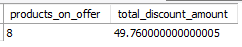Explanation

Aggregate function SUM helps in getting the total for input values. In the about, we want to calculate the number of products is on offer. First, we assign value 1 if a product is on an offer otherwise 0, then we are summing the 1’s to find the total count of products on offer.

In the second part of the aggregation, we want to calculate the total discount amount. Based on the condition on MRP and Selling price, we calculate the discount amount for each product, and then we are summing up using the SUM function.

Scenario 12: Create a variable with multiple values based on conditions and aggregate values for each level

For example, there are different value for payment mode, we want to assign a label to each of the mode value.

SQL Code:

`SELECT`

`CASE WHEN payment_mode=1 THEN 'Cash'`

`           WHEN payment_mode=2 THEN 'Card'`

`           WHEN payment_mode=3 THEN  'Sodexo'`

`           WHEN payment_mode=4 THEN  'UPI'`

`           ELSE 'Other' `

`         END as payment_mode,`

`      COUNT(*) as payment_count`

`from order_payments`

`GROUP BY 1;`

OUTPUT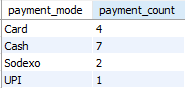Explanation

The case when statement can help us assign values to variable values based on a condition. In this example, the input variable payment_mode takes values 1 to 4, and the meaning of these values are 1 – Cash, 2- Card, 3-Sodexo, and 4-UPI. We need to assign these values and then count occurrences. We can group into identical values and then the count function gives the use count of rows for each of the payment_mode values.

Scenario 13: Find values/rows between two dates or two numeric values

For example, find orders that are of values between 100 and 200 amount.

SQL Code:

`SELECT * `

`FROM orders`

`WHERE amount BETWEEN 50 AND 100;`

OUTPUT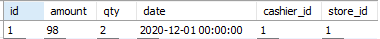Explanation

BETWEEN operator selects values within a given range.  In this example, we want to pull orders which has a value greater than and equal to 50 and less than equal to 100. This can be given using BETWEEN /AND

This can also be done using

`SQL CODE`

`SELECT * `

`FROM orders`

`WHERE amount >=50 `

`                AND AMOUNT<= 100;`

OUTPUTScenario 14: Selecting rows based on string value start with a particular character

For example, find employees where employee names are starting with M.

SQL Code:

`SELECT * `

`FROM employees`

`WHERE name LIKE "M%";`

OUTPUT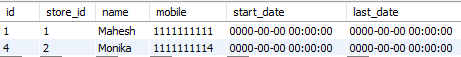Explanation

Character string value comparison is case sensitive. The LIKE operator in the WHERE clause can be used to search for a specified pattern.  In the above example, we are filtering employee names starting with “M”. % symbol indicates anything after M will be selected.

We can also find all the employees starting with any of the two characters and below is the code.

SQL Code

`select * `

`from employees`

`where (name like "M%") or (name like "S%");`

`;`

OUTPUT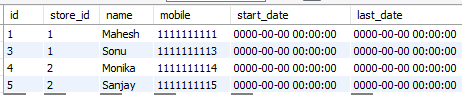Scenario 15: Finding all values where field containing a word

For example, we want to find all items (products) where the product name contains the “women” word.

We can do multiple ways.

SQL Code:

`SELECT * FROM items`

`WHERE UPPER(name) LIKE "%WOMEN%";`

OUTPUTExplanation

We can use % before and after a word to do a comparison of the string values.  But women can come with capital and small case. To not miss any of the relevant transactions, we can change the case to upper case using the UPPER () function before comparing the string values.

The `LIKE` the operator generally doesn't use any index and for the large size of the data, the query can be costly. If the text column is made as Full-Text Search.

`CREATE FULLTEXT INDEX `idx_items_name`  ON `dmart`.`items` (name) COMMENT '' ALGORITHM DEFAULT LOCK DEFAULT;`

When a column is defined as Full-Text Search and if we are using the below method of search a text string, it will be faster/more efficient than using LIKE with wildcarding.

`SELECT * FROM items WHERE MATCH(name)`

`AGAINST('women' IN NATURAL LANGUAGE MODE)`

`;`

OUTPUTJoins in MYSQL or SQL

So, all the scenarios discussed are related to manipulations with a single table. In a real project, we need to get data from multiple tables. So, for example in the current retail scenario, we want to see the number of distinct orders for each of the products. We need to join, items, and order tables to get the information required.

A JOIN clause can be used to combine two or more tables, based on a related column or columns between input tables.   By default, the combining of rows from input tables are done based on Cartesian products – each row of one table is linked to the other table. If any joining condition is applied, it filters out the rows that are not meeting the conditions.

Input Table: Customers

`  select * from retail.customers;`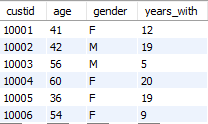Input Table: Products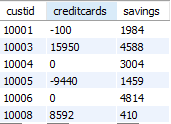Joining without any condition

`select * from retail.customers join  retail.products;`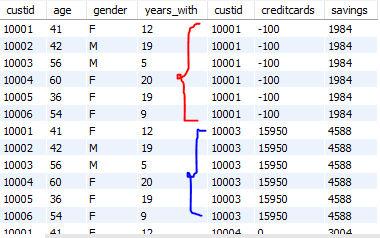Matching Tables based on a column

`select * from retail.customers c join `

`    retail.products p on c.custid=p.custid;`

OUTPUT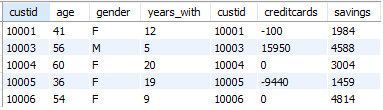The matching row condition is given using the ON clause. In both input tables, the custid column is available and can be used for matching the rows. If we do that 5 rows have the same custid values.

This join is called inner join or natural join. The above code is the same as

`SELECT  * FROM retail.customers A  inner join retail.Products B `

`                       ON A.custid =B.custid`

`                       ;`

What should we do if we want to keep all the custid value of table customer's table?

SQL Code:

`SELECT  * FROM retail.customers A  left join retail.Products B `

`                       ON A.custid =B.custid`

`                       ;`

OUTPUT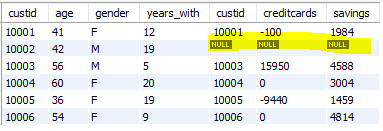This table for custid=1002 is not available in the products table but is still kept. This is called left join as it keeps all the rows of the table in the left even if not available in the other table.

What should we do if we want to keep all the custid value of table products table?

SQL Code

`SELECT  * FROM retail.customers A  right join retail.Products B `

`                       ON A.custid =B.custid`

`                       ;`

OUTPUT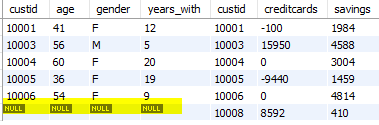Scenario 16: Finding item name, quantity, selling price, and the order number of all the items on a particular order id (say order id =5)

For example, in our scenario, the item name is available in the items table, and the order number is available in the order_items table, so we need to combine these tables and get all the information.

SQL Code:

`select b.order_id as 'Order NO',`

`        c.name as 'Product',`

`       b.qty as 'Quantity',`

`       b.selling_price as 'Selling Price'      `

`from order_items b,`

`     items c`

`where b.item_id = c.id`

`and      b.order_id=5;`

OUTPUT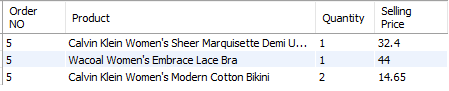Scenario 17: Retrieve data from multiple tables and aggregate data

For example, get Cashier Name when showing the count of orders by Cashiers

The cashier's name is available on the employee's table. We need to join the orders and employees table and then aggregate the details for each of the cashiers. Or we can aggregate or count orders for each of the cashiers and then get the names by joining with employees table.

SQL Code:

`select a.*, b.name as cashier_name`

`from `

`   (select cashier_id, count(*) as orders`

`    from orders`

`    group by 1`

`    )  a `

`    left join `

`    employees b`

`    on a.cashier_id = b.id`

`;`

OUTPUT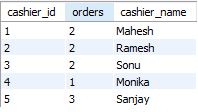Scenario 18: Group by multiple columns and get the summary statistics

For example, get order summary statistics by store and cashier.

we can aggregate the data by multiple columns. In this scenario, we need to aggregate first by store and then by cashiers. Also, we need to calculate the number of orders and the total value of these orders as well.

SQL Code:

`select s.name as store,`

`       c.name as Cashier,`

`       sum(o.amount) as amount,`

`       sum(o.qty) as qnty,`

`       count(*) as orders`

`from orders o inner join `

`     employees c    on o.cashier_id=c.id `

`     inner join `

`     stores s      on o.store_id=s.id`

`group by 1,2     `

`;`

OUTPUT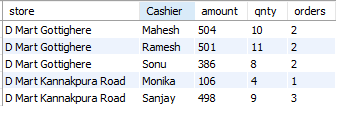Scenario 19: Create new aggregate tables

For example, we want to get the aggregated data stored in a table and apply variable transformations to the order quantity columns.

SQL Code:

`create temporary table tm_store_cashier_orders as `

`select s.name as store,`

`       c.name as Cashier,`

`       sum(o.amount) as amount,`

`       sum(o.qty) as qnty,`

`       count(*) as orders`

`from orders o inner join `

`     employees c    on o.cashier_id=c.id `

`     inner join `

`     stores s      on o.store_id=s.id`

`group by 1,2     `

`;`

`select * from tm_store_cashier_orders;`

OUTPUT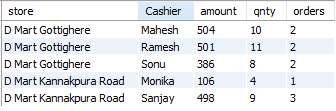Explanation

We can create a new table using CREATE clause. In the above code, we are creating a TEMPORARY table. A temporary table is a special type of table that allows you to store a temporary result. So, we can use queries to manipulate the data.

Scenario 20: Mathematical Transformations using SQL

For example, we may want to find log and square root transformations of order quantity.

These transformations can help in improving the accuracy of the machine learning models.

SQL Code:

`select a.*,`

`      log(qnty) as log_qnty,`

`      sqrt(qnty) as sqrt_qnty`

`from tm_store_cashier_orders a;`

OUTPUT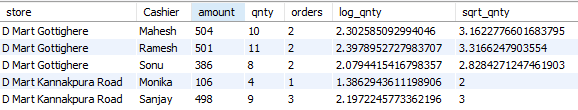We can round off the values and using the round() function.

`select a.*,`

`      round(log(qnty),2) as log_qnty,`

`      round(sqrt(qnty),2) as sqrt_qnty`

`from tm_store_cashier_orders a;`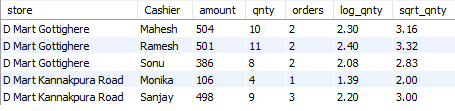Scenario 21: String Manipulations – Extracting Part of the String

For example, we want to extract the location of the store from the name.

SQL Code:

`select a.*,`

`       substr(store, 8, length(store)) as location`

`from tm_store_cashier_orders a;`

OUTPUT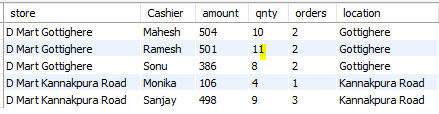Explanation

The length function helps us get the number of characters of the string.  And substr() helps us extract part of the input string based on starting position and the last string position.

Tags
Most PopularJun 18, 2020Jul 23, 2020Jun 19, 2020Jun 19, 2021